Engineering ToolBox - Resources, Tools and Basic Information for Engineering and Design of Technical Applications!

# Center of Gravity

## A body and the center of gravity.

The "center of gravity" for a volume, an area or a line - is the point at which the body - if suspended - would be in balance.

For a symmetrical body in a uniform material - the center of gravity would be in the geometric center.

### Perimeter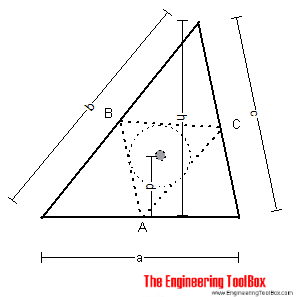The center of gravity is the center of the circle inscribed in triangle ABC (middle points of the sides of the triangle).

The distance d can be calculated as

d = h (b + c) / 2 (a + b + c)                                (1)

### Triangle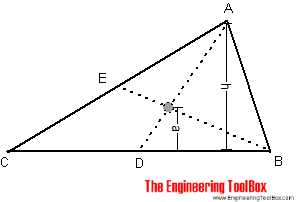The center of gravity of a triangle is at the intersection of lines BE and AD. The distance a can be calculated as

a = h / 3                               (2)

### Parallelogram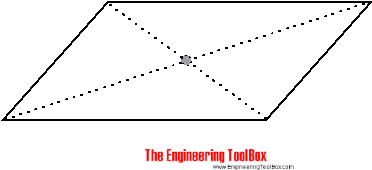The center of gravity of a parallelogram is at the intersection of the diagonals.

### Trapezoid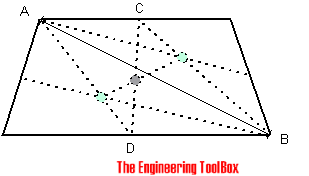The center of gravity of a trapezoid can be estimated by dividing the trapezoid in two triangles. The center of gravity will be in the intersection between the middle line CD and the line between the triangles centers of gravity.

### Two Bodies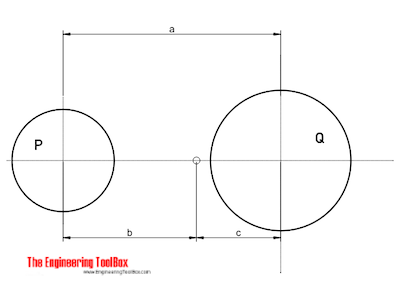The center of gravity of two bodies can be calculated as

b = Q a / (P + Q)                     (3a)

c = P a / (P + Q)                     (3b)

where

Q, P = weight or mass of the bodies (N, kg, lb, slugs)

## Related Topics

• Mechanics - Forces, acceleration, displacement, vectors, motion, momentum, energy of objects and more.
• Statics - Loads - forces and torque, beams and columns.
• Miscellaneous - Engineering related topics like Beaufort Wind Scale, CE-marking, drawing standards and more.
• Mathematics - Mathematical rules and laws - numbers, areas, volumes, exponents, trigonometric functions and more.

## Engineering ToolBox - SketchUp Extension - Online 3D modeling!

Add standard and customized parametric components - like flange beams, lumbers, piping, stairs and more - to your Sketchup model with the Engineering ToolBox - SketchUp Extension - enabled for use with the amazing, fun and free SketchUp Make and SketchUp Pro .Add the Engineering ToolBox extension to your SketchUp from the SketchUp Pro Sketchup Extension Warehouse!

Translate

## Privacy

We don't collect information from our users. Only emails and answers are saved in our archive. Cookies are only used in the browser to improve user experience.

Some of our calculators and applications let you save application data to your local computer. These applications will - due to browser restrictions - send data between your browser and our server. We don't save this data.

## Citation

• Engineering ToolBox, (2008). Center of Gravity. [online] Available at: https://www.engineeringtoolbox.com/center-gravity-d_1310.html [Accessed Day Mo. Year].

Modify access date.

. .

#### Scientific Online Calculator3 10